# sampling

(redirected from percutaneous blood sampling)
Also found in: Dictionary, Thesaurus, Medical, Financial.
Related to percutaneous blood sampling: chorionic villus sampling

## sampling

[′sam·pliŋ]
(engineering)
Process of obtaining a sequence of instantaneous values of a wave.
(science and technology)
The obtaining of small representative quantities of materials (gas, liquid, solid) for the purpose of analysis.
(statistics)
A drawing of a collection from a given population.

## Sampling

a statistical method of investigating the general properties of a set of some objects on the basis of a study of the properties of only part of these objects taken as a sample. The mathematical theory of sampling rests on two important branches of mathematical statistics—the theory of sampling from a finite set and the theory of sampling from an infinite set. The main distinction between sampling finite and infinite sets is that in the former case the sampling method is generally applied to objects of a nonrandom, determined nature (for instance, the number of defective products in a given batch of finished products is not a random quantity but an unknown constant that should be evaluated by the sample data). In the latter case, a sampling is usually used to study the properties of random events (for instance, to investigate the properties of continuously distributed random errors of measurements each of which in theory may be interpreted as the realization of one of an infinite set of possible results).

Sampling from finite set Sampling from a finite set and its theory are the foundation for statistical methods of quality control and are often used in sociological studies. According to probability theory, a sample will correctly reflect the properties of an entire population if the sampling is conducted at random—that is, in such a way that any of the possible samples of given size n from a population of size N [the number of such samples is equal to Nl/n\(N — n)l] has an identical probability of actually being selected.

In practice, the method used most often is sampling without replacement (nonrepeated sampling), in which each selected object is not returned to the set under investigation before the next object is chosen (such sampling is used in statistical quality control). Sampling with replacement (repeated sampling) is usually considered only in theoretical investigations (an example of repeated sampling is the registration of the number of particles touching in a certain period of time the walls of a vessel within which Brownian motion is under way). If n«N, then repeated and nonrepeated sampling yield practically equivalent results.

The properties of a set studied by the sampling method may be qualitative or quantitative. In the former case, the task of the sample survey is to determine the number M of objects in a set possessing some attribute (for instance, in statistical control one is often interested in the number M of defective products in a consignment of volume N). The ratio μN/n, where μ, is the number of objects with the given attribute in a sample of size n, may serve as an estimate of M. If the attribute is quantitative, then the task is to determine the mean value of the population = (x1 + x2 + … + xN)/N. An estimate of is the sampling mean ξ̄ = (ξ1 + ξ2 + … + ξ)/n, where ξ1 , … , ξn are those values from the investigated set x1, x2, … , xn that belong to the sample. From a mathematical standpoint, the first case is a particular variety of the second, which takes place when M of the quantities xi is equal to 1 and the other (N — M) are equal to 0; in this situation, x̄ = M/N and ξ̄ = μ/n

In the mathematical theory of sampling, the estimation of mean values occupies the central position because to a certain extent the study of the variation of an attribute within the set reduces to it, since the dispersion is usually taken as a measure of variation:The dispersion represents the mean value of the squares of the deviations x1 from their mean value . If a qualitative attribute is under study, σ2 = M(N - M)/N2.

The accuracy of the estimates μ/n and ξ̄ may be judged by their dispersions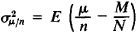and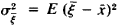which in terms of the dispersion of the finite set σ2 are expressed in the form of the ratios σ2/n in the case of repeated samples and σ2(N - n)/n(N - 1) in the case of unrepeated samples. Since in many problems of practical interest the random quantities μ/n and ξ conform, when n ≥ 30, to an approximately normal distribution, deviations of μ/n from which exceed 2σμ/n and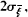respectively, in absolute value may, when n ≥ 30, occur in approximately one case out of 20 on the average. More complete information on the distribution of a quantitative attribute in a given population may be obtained by using the empirical distribution of this attribute in the sample.

Sampling from an infinite set In mathematical statistics the results of some uniform observations (most often indepen-dent) are commonly called a sample even when these results do not correspond to the concept of a repeated or non-repeated sampling from a finite set. For instance, the results of angular measurements of terrain, which are subject to independent continuously distributed random errors, are often called a sample from an infinite set. It is assumed that in principle any number of such observations may be made. The results actually obtained are considered a sample from an infinite set of possible results called a general set.

The concept of a general set is not logically irreproachable and necessary. In order to solve practical problems, an infinite general set itself is not needed but only various characteristics that are set in correspondence with it. From the standpoint of probability theory, these characteristics are numerical or functional characteristics of some probability distribution, and the sample units are random quantities that conform to this distribution. This interpretation makes it possible to extend to sampling estimates the general theory of statistical estimates.

It is for this reason, for instance, that in the probability theory of data processing the concept of an infinite general set is replaced by the concept of probability distribution, containing unknown parameters. The results of observations are interpreted as the experimentally observed values of the random quantities conforming to this distribution. The purpose of the processing is to compute, on the basis of the results of observations, statistical estimates that are in some sense optimal for the unknown parameters of distribution.

### REFERENCES

Dunin-Barkovskii, I. V., and N. V. Smirnov. Teoriia veroiatnostei i matematicheskaia statistika v tekhnike (Obshchaia chast’). Moscow, 1955. Chapter 5.
Kendall, M., and A. Stewart. Teoriia raspredelenii. Moscow, 1966. (Translated from English.)

L. N. BOL’SHEV

## sampling

(DSP)
The process of taking a sample of a signal at evenly spaced intervals of time. This is the first step in Digital Signal Processing.

## sampling

(1) In statistics, the analysis of a group by determining the characteristics of a significant percentage of its members chosen at random.

(2) Converting analog signals into digital form. Audio and other analog signals are continuous waveforms that are analyzed at various points in time and converted into digital samples. The accuracy with which the digital samples reflect their analog origins is based on "sampling rate" and "sample size." See A/D converter.

Sampling Rate - When to Measure
The sampling rate is the number of times per second that the waveform is measured, which typically ranges from 8 to 192 thousand times per second (8 kHz to 192 kHz). The greater the rate, the higher the frequency that can be captured. For a comparison of high-quality samples, see high-resolution sampling rates.

The sampling rate must be at least twice that of the analog frequency being captured. For example, the sampling rate used to create the digital data on a CD is 44.1 kHz, slightly more than double the 20kHz frequency an average person can hear. The sampling rate for digitizing voice for a toll-quality conversation is typically 8,000 times per second (8 kHz), twice the 4 kHz required for the full spectrum of the human voice. See analog and Nyquist theorem.

Sample Size - The Measurement
Also called "resolution" and "precision," the sample size is the measurement of each sample point on a numeric scale. Known as "quantizing," the sample point is turned into the closest whole number. The more granular the scale (the more increments), the more accurate the digital sample represents the original analog signal. See oversampling, quantization and PCM.

Sampling Sound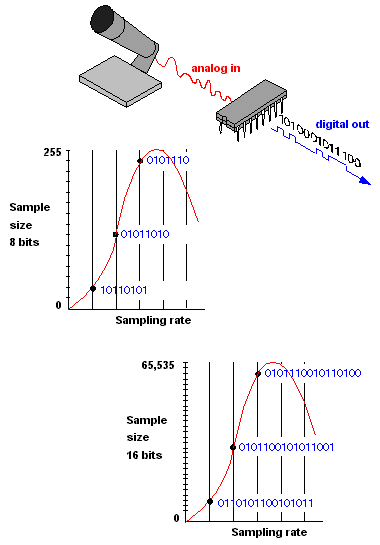The faster the sampling rate and the larger the sample size, the more accurately sound can be digitized. An 8-bit sample breaks the sound wave into 255 increments compared with 65,535 for a 16-bit sample.

Sampling Dialog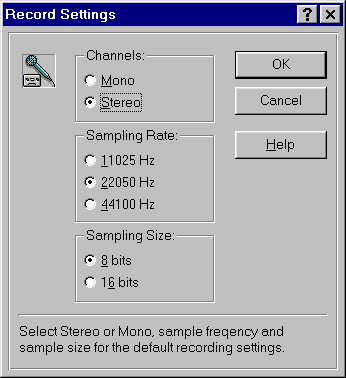This recording dialog from an earlier Sound Blaster sound card shows typical sampling options for digitizing sound into Windows WAV files.

DSD - A High-Res Sampling TechniqueDirect Stream Digital (DSD) is a dramatic departure from PCM. Instead of turning samples into a number with a range of values, DSD samples are only 1-bit long (0 or 1), depending on whether the wave is moving up or down from the previous sample point (see DSD).
Site: Follow: Share:
Open / Close